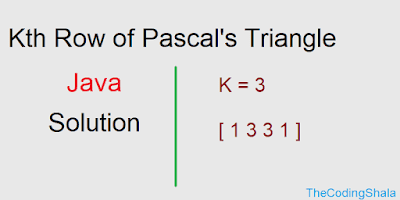## Posts

Showing posts from May, 2019

### Triplets with Sum between given range - The Coding ShalaHome >> Interview Questions >> Triplets with sum between given range Triplets with Sum between given range Java Solution Given an array of real numbers greater than zero in the form of strings. Find if there exists a  triplet  (a,b,c) such that  1  <  a+b+c  <  2  .  Return  1  for  true  or  0  for  false . Example: Given [0.6, 0.7, 0.8, 1.2, 0.4] , You should return  1 as 0.6+0.7+0.4=1.7 1<1.7<2 Hence, the output is 1. Triplets with Sum between given range Java Solution Here an array will be given, we need to find three values whose sum will be in the given range. We can solve this using brute force using three loops but that will give time limit error.  1 2 3 4 5 6 7 8 9 10 11 12 13 14 15 16 17 18 19 20 21 22 23 24 25 26 27 28 29 30 31 32 33 34 35 36 37 38 39 40 41 42 43 public class Solution { public int solve ( ArrayList < String > A ) { if ( A . size () < 3 )

### Anti Diagonals - The Coding ShalaHome >> Interview Questions >> Anti Diagonals Anti Diagonals Java Solution Give an N*N square matrix, return an array of its anti-diagonals. Look at the example for more details. Example: Input: 1 2 3 7 8 9 4 5 6 Return the following : [ , [2, 4], [3, 5, 7], [6, 8],  ] Input : 1 2 3 4 Return the following : [ , [2, 3],  ] Anti Diagonals Java Solution 1 2 3 4 5 6 7 8 9 10 11 12 13 14 15 16 17 18 19 20 21 22 23 24 25 26 27 28 29 30 31 public class Solution { public ArrayList < ArrayList < Integer >> diagonal ( ArrayList < ArrayList < Integer >> A ) { ArrayList < ArrayList < Integer >> ans = new ArrayList < ArrayList < Integer >>(); int N = A . size (); int i = N - 1 ; while ( i >= 0 ){ ArrayList < Integer > tmp = new ArrayList < Integer >();

### Kth Row of Pascal's Triangle - The Coding ShalaHome >> Interview Questions >> Kth row of pascal's triangle Kth Row of Pascal's Triangle Solution Java Given an index k, return the kth row of Pascal’s triangle. Example: Input : k = 3 Return : [1,3,3,1] Java Solution of Kth Row of Pascal's Triangle One simple method to get the Kth row of Pascal's Triangle is to generate Pascal Triangle till Kth row and return the last row.  1 2 3 4 5 6 7 8 9 10 11 12 13 14 15 public class Solution { public ArrayList < Integer > getRow ( int A ) { ArrayList < ArrayList < Integer >> res = new ArrayList < ArrayList < Integer >>(); for ( int i = 0 ; i <= A ; i ++){ ArrayList < Integer > tmp = new ArrayList < Integer >(); for ( int j = 0 ; j <= i ; j ++){ if ( j == i || j == 0 ) tmp . add ( 1 ); else tmp . add ( r